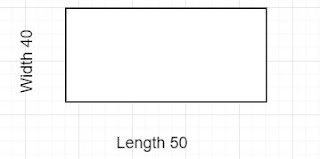# Square feet calculation of four unequal sides

Square feet calculation is straight forward if the sites have fixed length and width .See the example below and we use

In the above case we multiply length and width to compute the total square feet. So it will be 50*40=2000 square feet. Now if we have uneven numbers in all four side, How can we get the total square feet. We call this as Quadrilateral.

Just enter the below values  to calculate the total square feet of uneven sides

A-B -

A-D -

B-C -

C-D -

B-D - (Diagonal)

Total Square Feet isThanks for reading Square feet calculation of four unequal sides

Previous
« Prev Post